Skip to content

# CyanideCN/PyCINRAD

Decode CINRAD radar data and visualize.Fetching latest commit…
Cannot retrieve the latest commit at this time.
Type Name Latest commit message Commit time
Failed to load latest commit information.cinrad May 14, 2019example Jan 15, 2019pictures Apr 4, 2019test Apr 17, 2019ui Mar 14, 2019.gitattributes Sep 20, 2018.gitignore Apr 15, 2019.travis.yml Apr 18, 2019LICENSEREADME.md Apr 26, 2019README_zh.md Apr 26, 2019appveyor.yml Apr 18, 2019requirements.txt Apr 17, 2019requirements_dev.txt Apr 18, 2019setup.py May 12, 2019

# PyCINRADA python package which can read CINRAD radar data, perform calculations and visualize the data.

## Installation

``````pip install cinrad
``````

Python 3.5 +

Cartopy

Metpy

Shapefile

Pyresample

You can download from github page and then excecute

``````python setup.py install
``````

## Modules

### cinrad.datastruct

This submodule contains data structure used in this program.

Radial type data: `cinrad.datastruct.Radial`

Cross-section type data: `cinrad.datastruct.Slice_`

Grid type data: `cinrad.datastruct.Grid`

### cinrad.io

Decode CINRAD radar data.

Simple demonstration:

```from cinrad.io import CinradReader, StandardData
f = CinradReader(your_radar_file) #Old version data
f = StandardData(your_radar_file) #New standard data
f.get_data(tilt, drange, dtype) #Get data
f.get_raw(tilt, drange, dtype)```

The `get_raw` method returns radar records without other geographic information.

#### Saving data in NetCDF format

`f.to_nc(path_to_nc_file)`

#### Decode PUP data and SWAN data

`cinrad.io.PUP` provides functions to decode PUP data. The extracted data can be further used to create PPI. (Only radial data are supported.)

`cinrad.io.SWAN` provides similar interface to decode SWAN data.

```from cinrad.io import PUP
f = PUP(your_radar_file)
data = f.get_data()```

### cinrad.utils

This submodule provides some useful algorithms in radar meteorology. All functions contained only accept `numpy.ndarray`. This submodule extends the usage of this program, as these functions can accept customized data rather than only the data decoded by `cinrad.io`.

### cinrad.easycalc

For directly computation of decoded data, `cinrad.easycalc` provides functions that simplify the process of calculation. For functions contained in this submodule, only a list of reflectivity data is required as the argument.

Code to generate the required list:

`r_list = [f.get_data(i, 230, 'REF') for i in f.angleindex_r]`

#### VCS

`cinrad.easycalc.VCS` provides calculation of vertical cross-section for all variables.

```import cinrad
from cinrad.visualize import Section
f = cinrad.io.CinradReader(your_radar_file)
rl = [f.get_data(i, 230, 'REF') for i in f.angleindex_r]
vcs = cinrad.easycalc.VCS(rl)
sec = vcs.get_section(start_cart=(111, 25.5), end_cart=(112, 26.7)) # pass geographic coordinates (longitude, latitude)
sec = vcs.get_section(start_polar=(115, 350), end_polar=(130, 30)) # pass polar coordinates (distance, azimuth)
fig = Section(sec)
fig('D:\\')```

### cinrad.correct

This submodule provides algorithms to correct raw radar fields.

#### cinrad.correct.dealias

This function can unwrap the folded velocity using algorithm originated from `pyart`. (needs C compiler)

```import cinrad
#(some codes omitted)
v = f.get_data(1, 230, 'VEL')
v_corrected = cinrad.correct.dealias(v)```

### cinrad.visualize

Visualize the data stored in acceptable format (`cinrad.datastruct`). It also means that you can using customized data to construct a object belongs to one of the class in `cinrad.datastruct` and then perform visualization. For further information about this method, please see the examples contained in `example` folder.

Simple demonstration:

```from cinrad.visualize import PPI
fig = PPI(R) #Plot PPI
fig('D:\\') #Pass the path to save the fig
from cinrad.visualize import Section
fig = Section(Slice_) #Plot VCS
fig('D:\\')
from cinrad.visualize import RHI
fig = RHI(rhi) #Plot data from RHI scan mode
fig('D:\\')```

The path passed into the class can either be the folder path or the file path. Also, if no path is passed, the figure will be saved at the folder named `PyCINRAD` in the home folder (e.g. `C:\Users\tom`).

#### Customize plot settings

The summary of args that can be passed into `PPI` are listed as follows.

arg function
`cmap` colormaps used for plotting
`norm` norm used for plotting
`nlabel` number of labels on the colorbar
`label` labels on the colorbar
`highlight` highlight area of input name
`dpi` dpi of figure
`extent` area to plot e.g. `extent=[90, 91, 29, 30]`
`add_slice` add cross-section data to ppi plot
`style` control the background color `black` or `white`
`add_city_names` annotate name of city on the plot

Besides args, class `PPI` has some other auxiliary plotting functions.

##### PPI.plot_range_rings(self, _range, color='white', linewidth=0.5, **kwargs)

Plot range rings on the PPI plot.

##### PPI.plot_cross_section(self, data, ymax=None)

Plot VCS section under the PPI plot.

This function is very similar to `vcs` argument of `PPI`, but the range of y-axis can be adjusted only by this function.

##### PPI.storm_track_info(self, filepath)

Plot PUP STI product on the current PPI map, including past position., current position, and forecast positions.

## Gallery

#### PPI reflectivity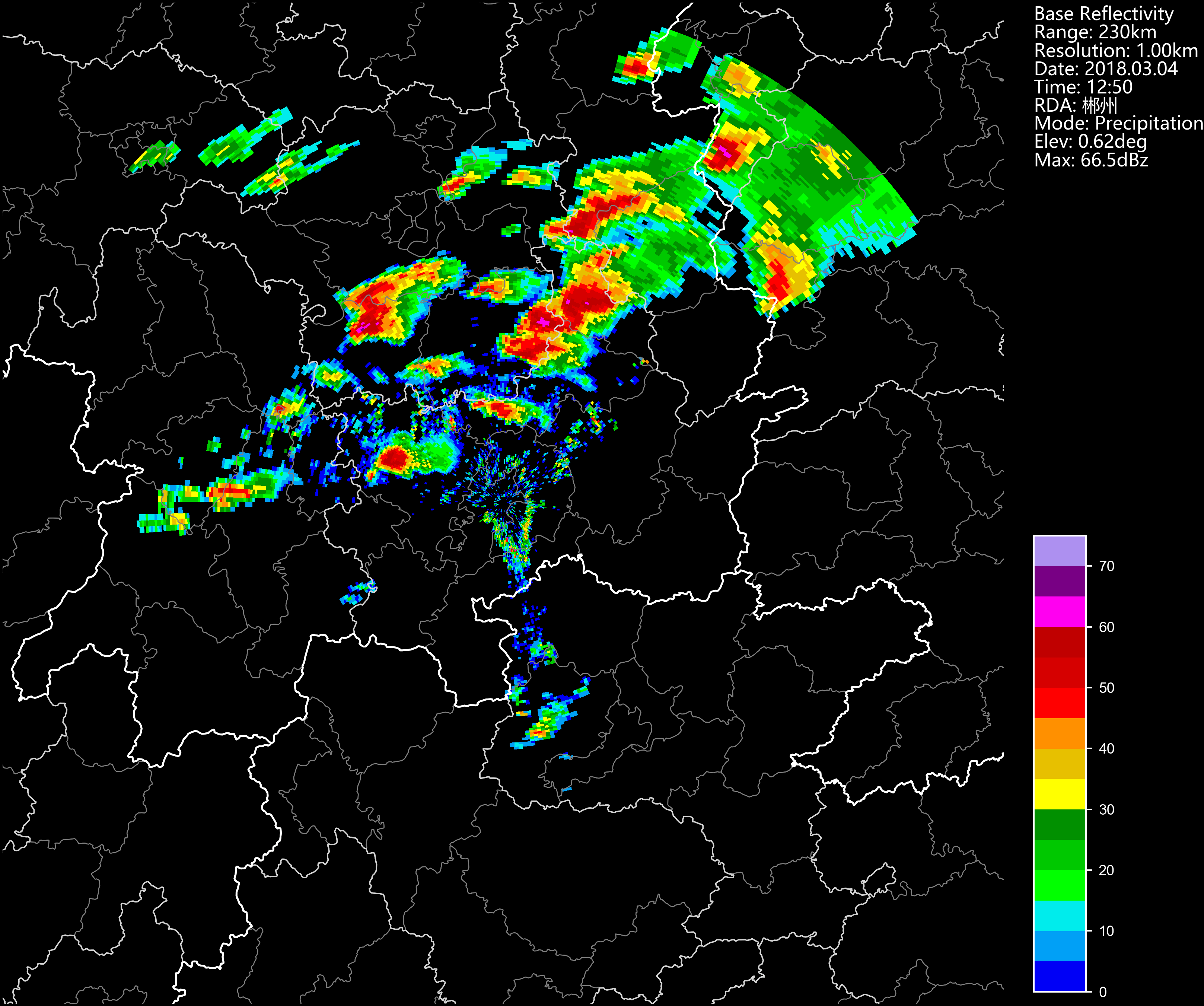#### PPI reflectivity combined with cross-section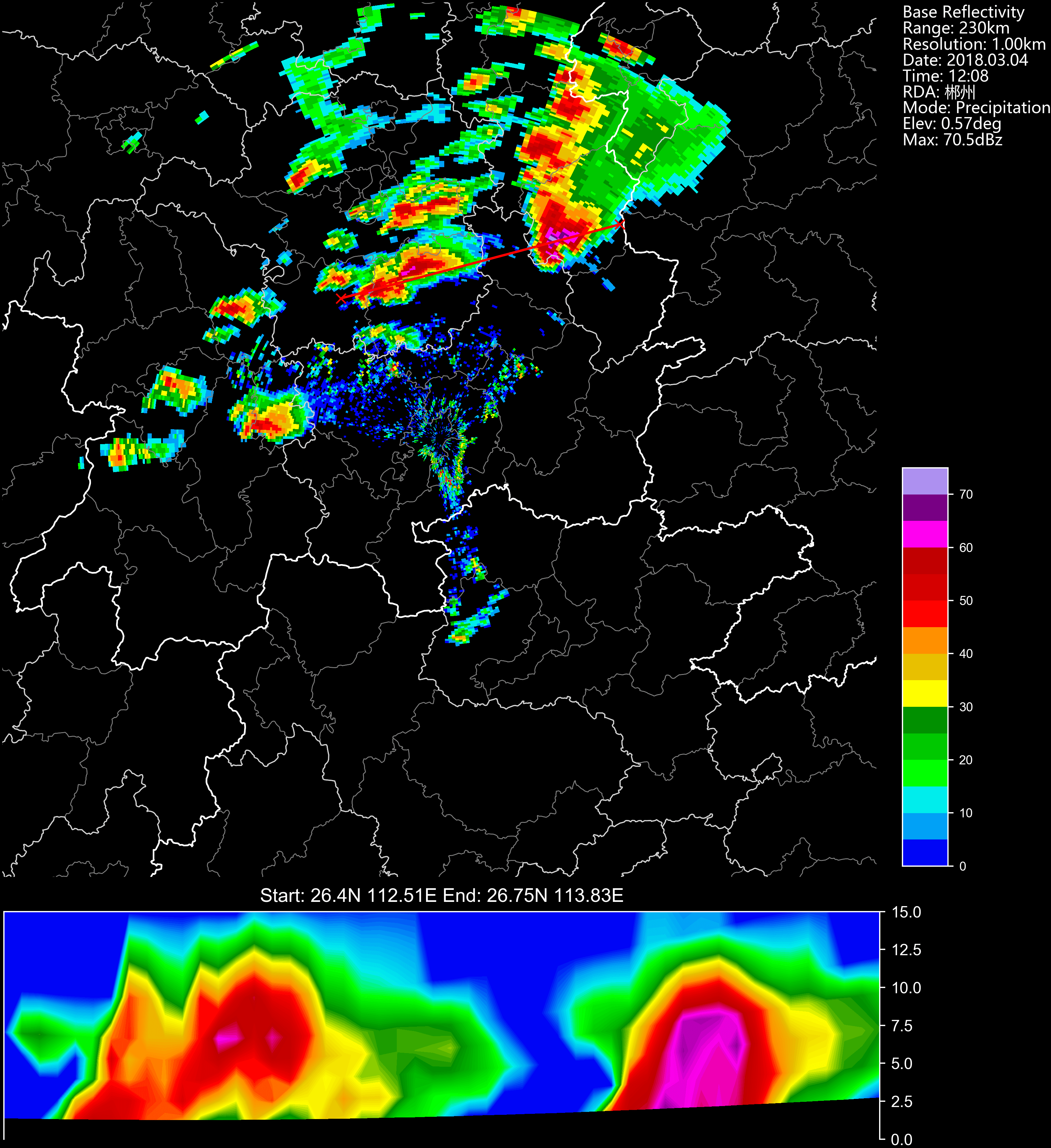#### Cross-section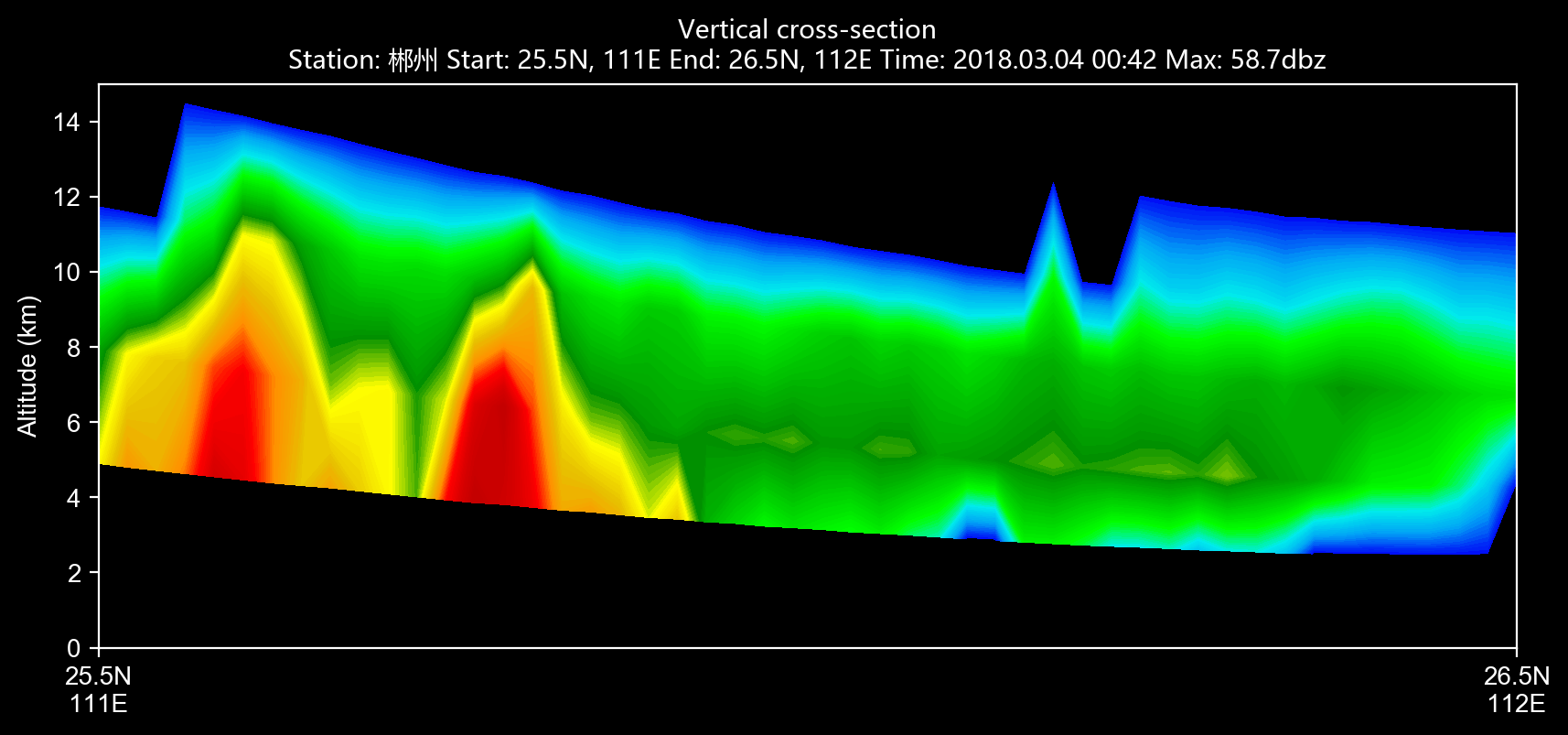#### Cross-section other than reflectivity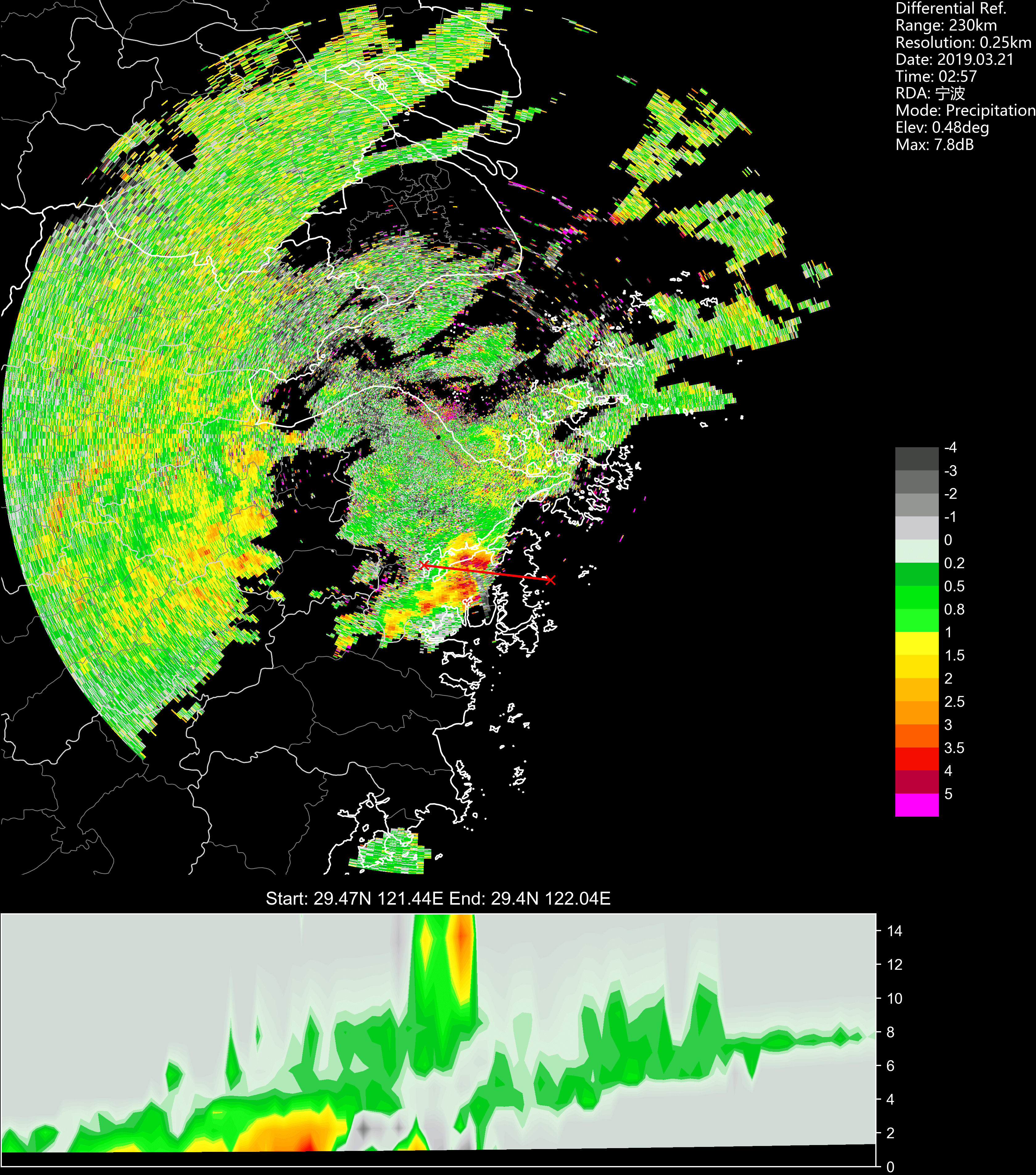#### RHI reflectivity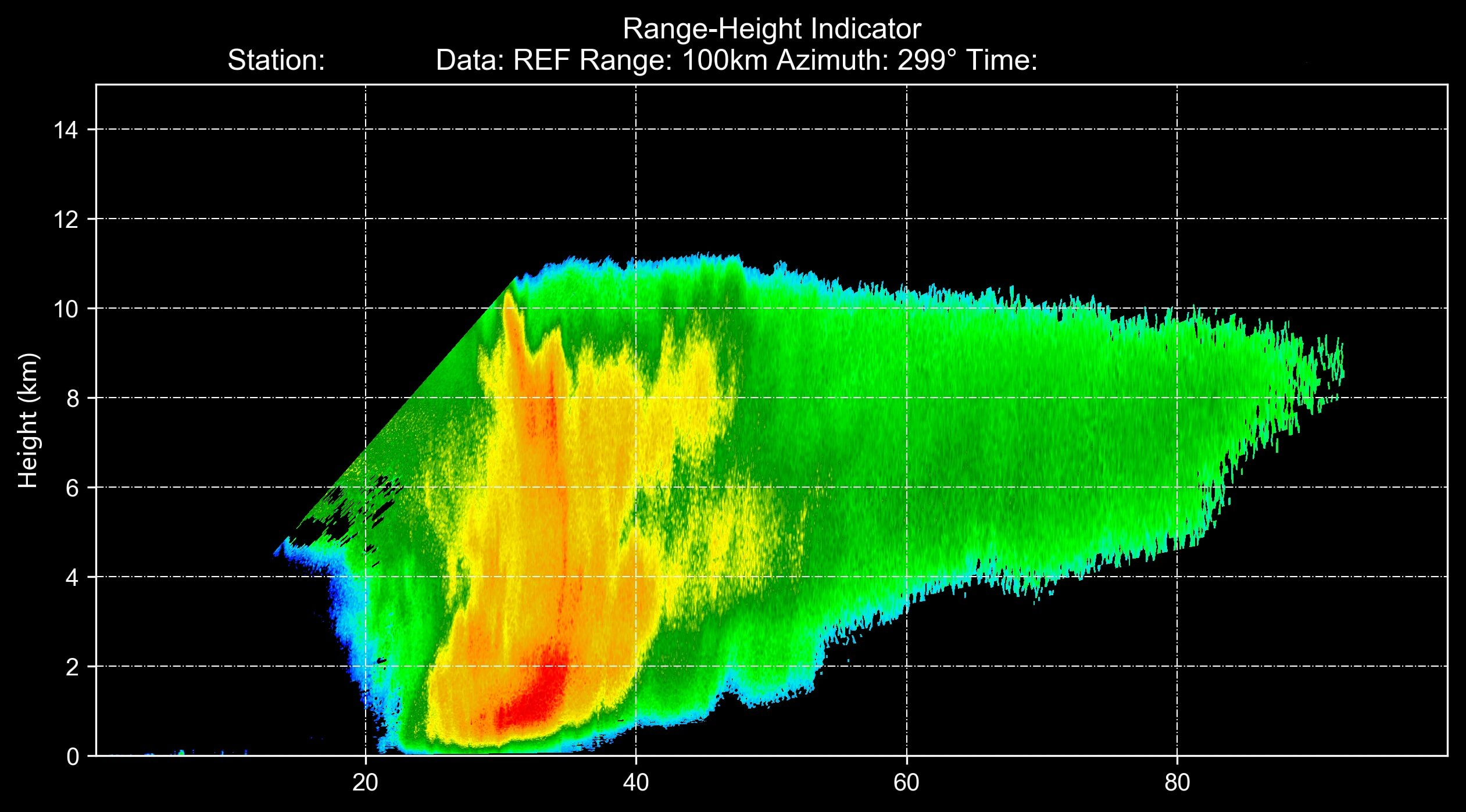## Notes

If you are interested in this program, you can join the developers of this program. Any contribution is appreciated!

If you have some questions or advise about this program, you can create a issue or email me at 274555447@qq.com.

You can’t perform that action at this time.